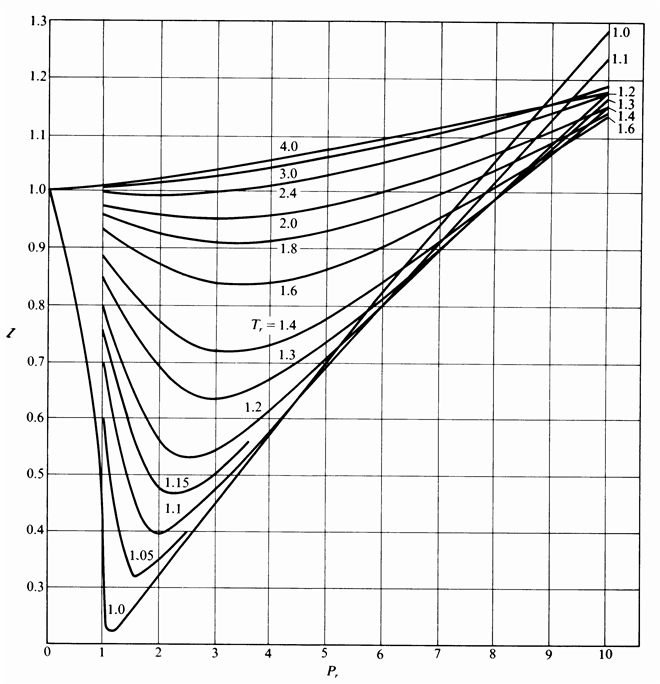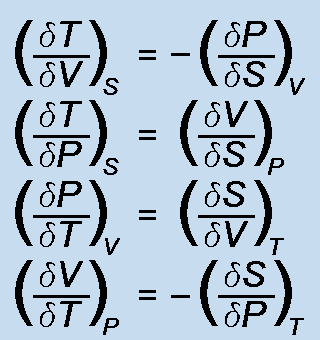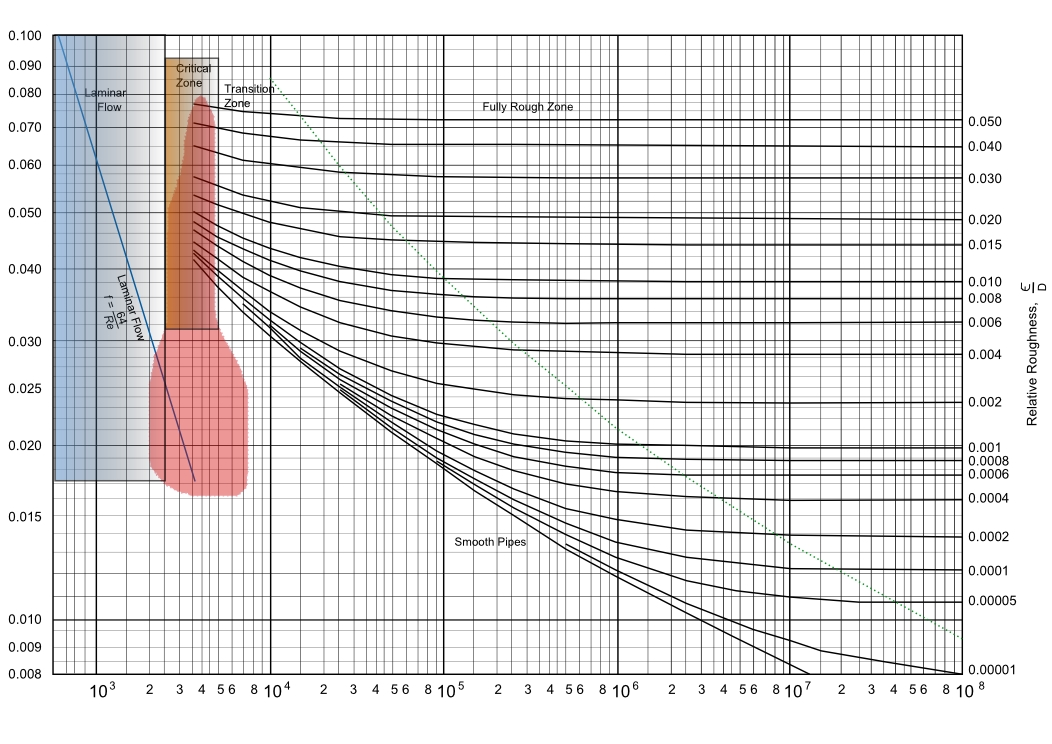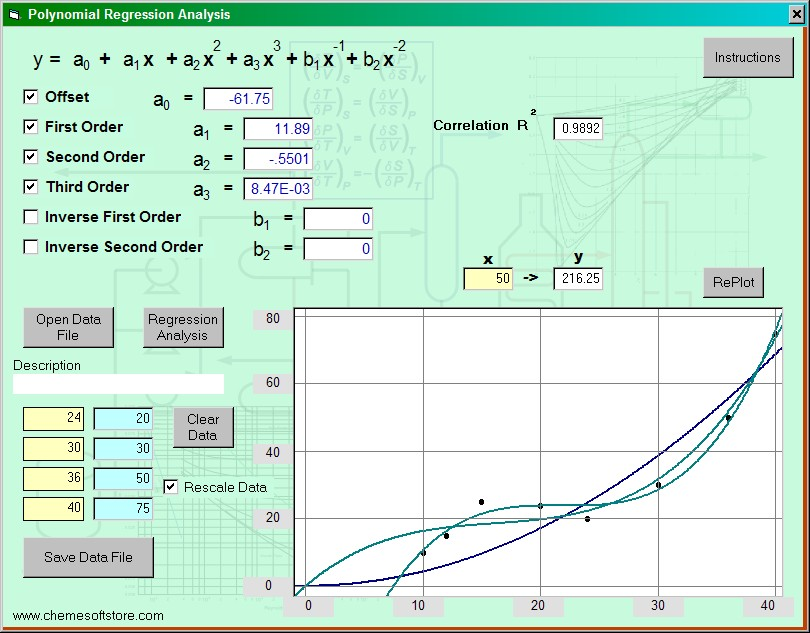### ChemE Soft StoreCaCO3 -> CaO + CO2
NaCl + NH3 + CO2 + H2O -> NaHCO3 + NH4Cl
2 NaHCO3 -> Na2 CO3 + H2O + CO2
CaO + H2 O -> Ca(OH)2
Ca(OH)2 + 2 NH4 Cl -> CaCl2 + 2 NH3 + 2 H2O

## Polynomial Regression

Polynomial regression analysis of x-y data for six orders of the polynomial equation. Specified orders are solved, plotted, and correlation coefficients calculated.#### Features:

• Orders of the polynomial equation can be chosen for any combination of the six orders
• Correlation coefficients for the chosen equation is calculated and shown
• Equations can be plotted for visual comparison
• Y value for the chosen polynomial equation can be calculated and shown
• Instructions for the software application is presented